# Hermitian matrix

Hermitian-symmetric matrix, self-conjugate matrix

A square matrix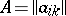overthat is the same as its Hermitian-conjugate matrix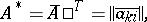that is, a matrix whose entries satisfy the condition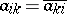. If all the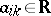, then a Hermitian matrix is symmetric (cf. Symmetric matrix). The Hermitian matrices of a fixed order form a vector space over. Ifandare two Hermitian matrices of the same order, then so is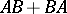. Under the operation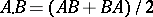the Hermitian matrices (of order) form a Jordan algebra. The productof two Hermitian matrices is itself Hermitian if and only ifandcommute.

The Hermitian matrices of orderare the matrices of Hermitian transformations of an-dimensional unitary space in an orthonormal basis (see Self-adjoint linear transformation). On the other hand, Hermitian matrices are the matrices of Hermitian forms in an-dimensional complex vector space. Like Hermitian forms (cf. Hermitian form), Hermitian matrices can be defined over any skew-field with an anti-involution.

All eigen values of a Hermitian matrix are real. For every Hermitian matrixthere exists a unitary matrixsuch thatis a real diagonal matrix. A Hermitian matrix is called non-negative (or positive semi-definite) if all its principal minors are non-negative, and positive definite if they are all positive. Non-negative (positive-definite) Hermitian matrices correspond to non-negative (positive-definite) Hermitian linear transformations and Hermitian forms.